# AP Physics 2 Practice Test 8

### Test Information10 questions18 minutes

1.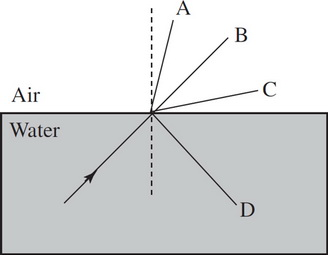A beam of light goes from water to air. Depending on the actual angle that the light strikes the surface, which of the following are possible outcomes?

2.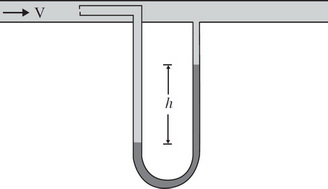The instrument in an aircraft to measure airspeed is known as the pitot tube, shown in the figure above. The opening facing the incoming air (with the small aperature) is the part meant to capture the air at rest. The opening perpendicular to the flow of air (with the large aperature) is meant to capture air at speed. If h = 1 m and the fluid within the manometer is water, what is the airspeed? Take the density of air to be rair = 1.2 kg/m3.

3. When a light wave in air hits a thin film (nfilm > nair), part of the wave is transmitted into the film and part is reflected. At the bottom of the thin film part of the wave is transmitted into air and part is reflected back up. What can you say about the reflected wave's phase at the top layer and bottom layers?

4. An object is placed 10 cm in front of a diverging mirror. What is the focal length of the mirror if the image appears 2 cm behind the mirror?

5.In the figure above, heat flows through a block of iron as shown in Figure A at a rate of H. The temperature on each side of the block are T1 and T2 respectively. If the block is rotated 90º as shown in Figure B, the rate at which heat is transferred is now

6. Two charged, massive particles are isolated from all influence except those between the particles. They have charge and mass such that the net force between them is 0 N. Which of the following is NOT true?

7. Question below refer to the following situation:

Uranium-238 decays into thorium-234 and an alpha particle as illustrated below.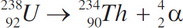What is the ratio of neutrons in thorium to the number of neutrons in uranium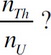8. Question below refer to the following situation:

Uranium-238 decays into thorium-234 and an alpha particle as illustrated below.Which of the following statements is NOT true for the above reaction?

9.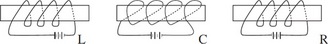Three solenoids are arranged next to each other as shown above. What can we say about the solenoids interactions?

10. A particle of mass m and charge Q and velocity V is directed toward a uniform electric field of strength E and travels a distance d. How far does the particle travel if the original velocity is doubled and the mass is cut in half?